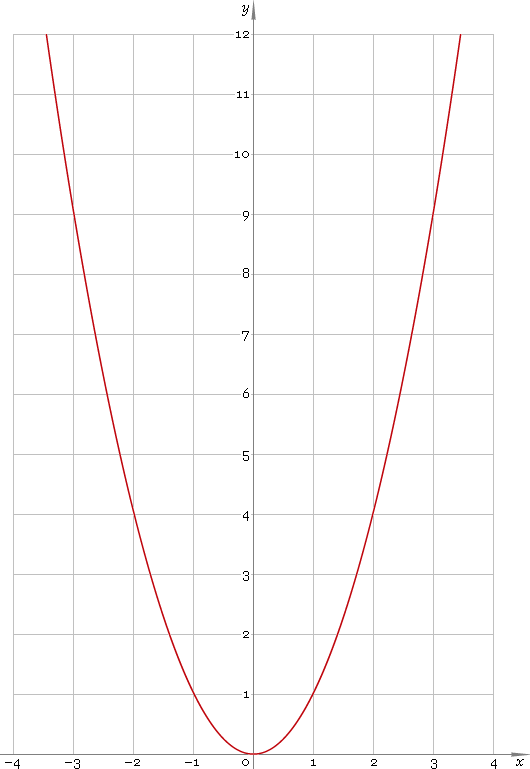The Art of Mathematics

# ^ — power function

## 1. Definition

Power function is the function of kind

xa

## 2. Graph

Power function domain depends on power a, but it is always defined for positive real half-axis (0, +∞). Parabola graph for a = 2 is depicted below — fig. 1.Fig. 1. Graph of the power function y = x2.

Function codomain as well depends on power a, but it always includes positive half of the real axis (0, +∞).

## 3. Identities

Negative power:

xa = 1 /xa

Power sum and difference:

xa + b = xa xb
xab = xa/xb

Power product:

xab = (xa)b

## 4. Derivative and indefinite integral

Power derivative:

xa′ = a xa−1

In particular:

(1 /x)′ = x−1′ = −x−2 = −1 /x2

Indefinite integral of the power:

xa dx = xa+1 / (a + 1) + C

for a ≠ −1, in the case a = −1:

∫ 1 /x dx = ln|x| + C

Here and above C is an arbitrary constant.

## 5. How to use

To calculate power of the number:

``9^2;``

To get power of the complex number:

``(9+i*7)^(2−i);``

To calculate power of the current result:

``rslt^2;``

To engage the current result as power:

``9^rslt;``

To get power of the number z in calculator memory:

``mem[z]^2;``

To engage number z in memory as power:

``9^mem[z];``

## 6. Support

Power function of the real argument is supported in free version of the Librow calculator.

Power function of the complex argument is supported in professional version of the Librow calculator.